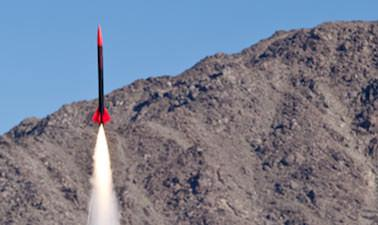# HarveyMuddX: How Stuff Moves, Part 3: Wave Motion

A Calculus-based introduction to Newtonian mechanics that emphasizes problem-solving.4 weeks
6 hours per week
Instructor-paced
Instructor-led on a course schedule
This course is archived

WHAT IS “HOW STUFF MOVES”?
Mechanics is the study of how things move. It was the first quantitative science to achieve wide power to predict behavior, including things never before directly observed. Newton, Leibniz, and others invented calculus to describe motion and we will find both differential and integral calculus extremely useful throughout this course.

This is the third in a 3-part series of courses that parallels the second-semester mechanics course taught at Harvey Mudd College. Part 3 focuses on the movement of oscillating systems and the propagation of waves (sound, seismic, or surface-water). Part 1 examined linear motion, and Part 2 examined angular motion. This course is an invitation to develop your problem-solving skills and to learn how to apply mathematics to all sorts of problems of the physical world. Learning the rules that govern how stuff moves in the world around us is exciting; using those rules to predict _correctly _something that you haven’t observed means that you really understand something. It‘s a great feeling.

WHAT SHOULD I KNOW BEFORE WE START?
You need not have taken physics before, but we assume that you have studied mathematics, up to and including a first course in calculus. You may be taking a calculus course concurrently with this course; that should be a good strategy. We will introduce important calculus ideas and methods as the need arises and provide examples.

There is a Mathematics Diagnostic Test that you can take at the beginning of Part 1 of this series to ensure that your mathematics background will set you up for success in this course.

### At a glance

• Institution: HarveyMuddX
• Subject: Physics
• Level: Introductory
• Prerequisites:
How Stuff Moves Parts 1 and 2.
• Language: English
• Video Transcript: English
• Associated skills: Mechanics, Calculus, Diagnostic Tests, Angular (Web Framework), Physics, Problem Solving, Integral Calculus, Linear Motion

# What you'll learn

Skip What you'll learn
• The basic physics of how objects move
• How to understand the movement of oscillating systems
• The mechanics of the propagation of waves (sound, seismic, or surface-water)"